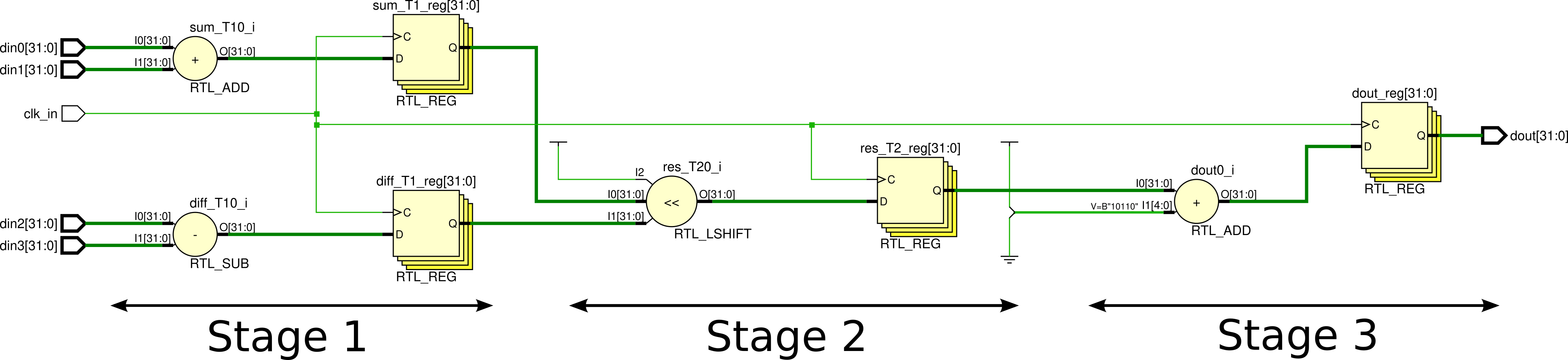## Pipeline using non-blocking assignment

Implements $$\{(A_0 + A_1) << (A_3-A_4)\} + 22$$ as three stage pipeline:

Stages:

1. Add $$A_0$$ to $$A_1$$, subtract $$A_4$$ from $$A_3$$ and assign the values to $$t_0$$ and $$t_1$$ respectively.
2. Left shift $$t_0$$ by $$t_1$$.
3. Add 22 to the result from stage 2.

Elaborated design:## Synthesis using for-loops

Pipeline implementation using for loops